# Model-based boosting in R: a hands-on tutorial using the R package mboost

## Abstract

We provide a detailed hands-on tutorial for the R add-on package mboost. The package implements boosting for optimizing general risk functions utilizing component-wise (penalized) least squares estimates as base-learners for fitting various kinds of generalized linear and generalized additive models to potentially high-dimensional data. We give a theoretical background and demonstrate how mboost can be used to fit interpretable models of different complexity. As an example we use mboost to predict the body fat based on anthropometric measurements throughout the tutorial.

This is a preview of subscription content, access via your institution.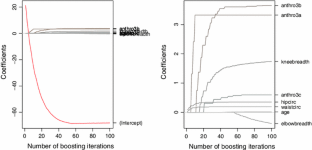Fig. 1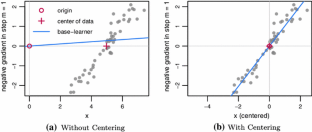Fig. 2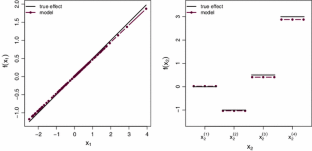Fig. 3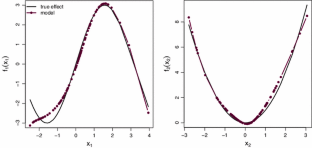Fig. 4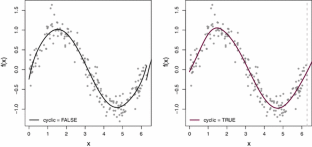Fig. 5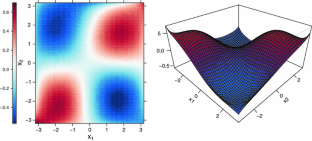Fig. 6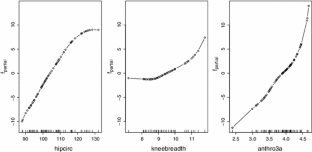Fig. 7Fig. 8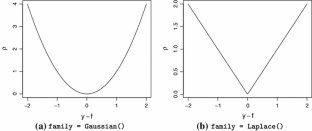Fig. 9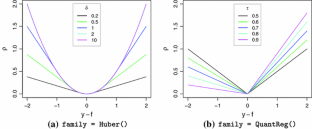Fig. 10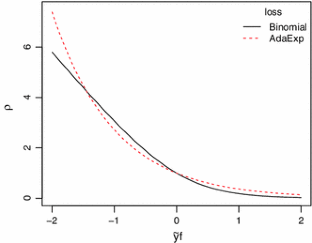Fig. 11
1. 1.

Note that here and in the following we sometimes restrict the focus to the most important or most interesting arguments of a function. Further arguments might exist. Thus, for a complete list of arguments and their description we refer to the respective manual.

2. 2.

glmboost() merely handles the preprocessing of the data. The actual fitting takes place in a unified framework in the function mboost_fit().

3. 3.

Another alternative is given by the matrix interface for glmboost() where one can directly use the design matrix as an argument. For details see ?glmboost.

4. 4.

If the fitting function glmboost() is used the base-learners never contain an intercept. Furthermore, linear base-learners without intercept can be obtained by specifying a base-learner bols(x, intercept = FALSE) (see below).

5. 5.

gamboost() also calls mboost_fit() for the actual boosting algorithm.

6. 6.

The name refers to ordinary least squares base-learner.

7. 7.

If df is specified in bols(), lambda is always ignored.

8. 8.

Until mboost 2.1-3 the default was trace($$\mathcal{S }$$), from version 2.2-0 onwards the default now is trace ($$2\mathcal{S }-\mathcal{S }^{T}\!\mathcal{S }$$).

9. 9.

The name refers to B-splines with penalty, hence the second b.

10. 10.

If lambda is specified in bbs(), df is always ignored.

11. 11.

Note that df = 4 was changed to df = 6 in mboost 2.1-0.

12. 12.

See ?AIC.boost for further details.

13. 13.

The percentage of observations to be included in the learning samples for subsampling can be specified using a further argument in cv() called prob. Per default this is 0.5.

14. 14.

Note that in mboost the response must be specified as a binary factor.

15. 15.

The unused weights argument w is required to exist by mboost when the function is (internally) called. It is hence ’specified’ as NULL.

## References

1. Bates D, Maechler M, Bolker B (2011) lme4: linear mixed-effects models using S4 classes. http://CRAN.R-project.org/package=lme4, R package version 0.999375-42

2. Breiman L (1998) Arcing classifiers (with discussion). Ann Stat 26:801–849

3. Breiman L (1999) Prediction games and arcing algorithms. Neural Comput 11:1493–1517

4. Breiman L (2001) Random forests. Mach Learn 45:5–32

5. Bühlmann P (2006) Boosting for high-dimensional linear models. Ann Stat 34:559–583

6. Bühlmann P, Hothorn T (2007) Boosting algorithms: regularization, prediction and model fitting (with discussion). Stat Sci 22:477–522

7. Bühlmann P, Yu B (2003) Boosting with the $$L_2$$ loss: regression and classification. J Am Stat Assoc 98: 324–338

8. de Boor C (1978) A practical guide to splines. Springer, New York

9. Efron B, Hastie T, Johnstone L, Tibshirani R (2004) Least angle regression. Ann Stat 32:407–499

10. Eilers PHC, Marx BD (1996) Flexible smoothing with B-splines and penalties (with discussion). Stat Sci 11:89–121

11. Fan J, Lv J (2010) A selective overview of variable selection in high dimensional feature space. Statistica Sinica 20:101–148

12. Fenske N, Kneib T, Hothorn T (2011) Identifying risk factors for severe childhood malnutrition by boosting additive quantile regression. J Am Stat Assoc 106(494):494–510

13. Freund Y, Schapire R (1996) Experiments with a new boosting algorithm. In: Proceedings of the thirteenth international conference on machine learning theory. Morgan Kaufmann, San Francisco, pp 148–156

14. Friedman JH (2001) Greedy function approximation: a gradient boosting machine. Ann Stat 29:1189–1232

15. Friedman JH, Hastie T, Tibshirani R (2000) Additive logistic regression: a statistical view of boosting (with discussion). Ann Stat 28:337–407

16. Garcia AL, Wagner K, Hothorn T, Koebnick C, Zunft HJF, Tippo U (2005) Improved prediction of body fat by measuring skinfold thickness, circumferences, and bone breadths. Obes Res 13(3):626–634

17. Hastie T (2007) Comment: Boosting algorithms: regularization, prediction and model fitting. Stat Sci 22:513–515

18. Hastie T, Tibshirani R (1990) Generalized additive models. Chapman & Hall, London

19. Hastie T, Tibshirani R, Friedman J (2009) The elements of statistical learning: data mining, inference, and prediction, 2nd edn. Springer, New York

20. Hofner B (2011) Boosting in structured additive models. PhD thesis, Department of Statistics, Ludwig-Maximilians-Universität München, Munich

21. Hofner B, Hothorn T, Kneib T, Schmid M (2011a) A framework for unbiased model selection based on boosting. J Comput Graph Stat 20:956–971

22. Hofner B, Müller J, Hothorn T (2011b) Monotonicity-constrained species distribution models. Ecology 92:1895–1901

23. Hothorn T, Hornik K, Zeileis A (2006) Unbiased recursive partitioning: a conditional inference framework. J Comput Graph Stat 15:651–674

24. Hothorn T, Bühlmann P, Kneib T, Schmid M, Hofner B (2010) Model-based boosting 2.0. J Mach Learn Res 11:2109–2113

25. Hothorn T, Bühlmann P, Kneib T, Schmid M, Hofner B (2012) mboost: model-based boosting. http://CRAN.R-project.org/package=mboost, R package version 2.1-3

26. Kneib T, Hothorn T, Tutz G (2009) Variable selection and model choice in geoadditive regression models. Biometrics 65:626–634. Web appendix accessed at http://www.biometrics.tibs.org/datasets/071127P.htm on 16 Apr 2012

27. Koenker R (2005) Quantile regression. Cambridge University Press, New York

28. Mayr A, Fenske N, Hofner B, Kneib T, Schmid M (2012a) Generalized additive models for location, scale and shape for high-dimensional data—a flexible approach based on boosting. J R Stat Soc Ser C (Appl Stat) 61(3):403–427

29. Mayr A, Hofner B, Schmid M (2012b) The importance of knowing when to stop—a sequential stopping rule for component-wise gradient boosting. Methods Inf Med 51(2):178–186

30. Mayr A, Hothorn T, Fenske N (2012c) Prediction intervals for future BMI values of individual children—a non-parametric approach by quantile boosting. BMC Med Res Methodol 12(6):1–13

31. McCullagh P, Nelder JA (1989) Generalized linear models, 2nd edn. Chapman & Hall, London

32. Meinshausen N (2006) Quantile regression forests. J Mach Learn Res 7:983–999

33. Pinheiro J, Bates D (2000) Mixed-effects models in S and S-PLUS. Springer, New York

34. Pinheiro J, Bates D, DebRoy S, Sarkar D, R Development Core Team (2012) nlme: linear and nonlinear mixed effects models. http://CRAN.R-project.org/package=nlme, R package version 3.1-103

35. R Development Core Team (2012) R: a language and Environment for statistical computing. R Foundation for Statistical Computing, Vienna. http://www.R-project.org, ISBN 3-900051-07-0

36. Ridgeway G (2010) gbm: generalized boosted regression models. http://CRAN.R-project.org/package=gbm, R package version 1.6-3.1

37. Schmid M, Hothorn T (2008a) Boosting additive models using component-wise P-splines. Comput Stat Data Anal 53:298–311

38. Schmid M, Hothorn T (2008b) Flexible boosting of accelerated failure time models. BMC Bioinform 9:269

39. Schmid M, Potapov S, Pfahlberg A, Hothorn T (2010) Estimation and regularization techniques for regression models with multidimensional prediction functions. Stat Comput 20:139–150

40. Schmid M, Hothorn T, Maloney KO, Weller DE, Potapov S (2011) Geoadditive regression modeling of stream biological condition. Environ Ecol Stat 18(4):709–733

41. Sobotka F, Kneib T (2010) Geoadditive expectile regression. Comput Stat Data Anal 56(4):755–767

42. Tierney L, Rossini AJ, Li N, Sevcikova H (2011) snow: simple network of workstations. http://CRAN.R-project.org/package=snow, R package version 0.3-7

43. Urbanek S (2011) multicore: parallel processing of R code on machines with multiple cores or CPUs. http://CRAN.R-project.org/package=multicore, R package version 0.1-7

Download references

## Acknowledgments

The authors thank two anonymous referees for their comments that helped to improve this article.

## Author information

Authors

### Corresponding author

Correspondence to Benjamin Hofner.

## Appendix: Building your own family

### Appendix: Building your own family

Via the constructor function Family(), in mboost there exists an easy way for the user to set up new families. The main required arguments are the loss to be minimized and the negative gradient (ngradient) of the loss. The risk is then commonly defined as the sum of the loss over all observations.

We will demonstrate the usage of this function by (re-) implementing the family to fit quantile regression (the pre-defined family is QuantReg()). In contrast to standard regression analysis, quantile regression (Koenker 2005) does not estimate the conditional expectation of the conditional distribution but the conditional quantiles. Estimation is carried out by minimizing the check function $$\rho _{\tau }(\cdot )$$:

\begin{aligned} \rho _{\tau }(y_i - f_{\tau i} ) = \left\{ \begin{array}{ll} (y_i - f_{\tau i} ) \cdot \tau&\quad (y_i - f_{\tau i} ) \ge 0 \\ (y_i - f_{\tau i} ) \cdot (\tau -1)&\quad (y_i - f_{\tau i} ) <0, \end{array} \right. \end{aligned}

which is depicted in Fig. 10b. The loss for our new family is therefore given as:

The check-function is not differentiable at the point 0. However in practice, as the response is continuous, we can ignore this by defining:

\begin{aligned} - \frac{\partial \rho _{\tau }(y_i, f_{\tau i})}{\partial f} = \left\{ \begin{array}{l@{\quad }l} \tau&(y_i - f_{\tau i}) \ge 0 \\ \tau -1&(y_i - f_{\tau i}) <0. \end{array} \right. \end{aligned}

The negative gradient of our loss is therefore:Footnote 15

Of further interest is also the starting value for the algorithm, which is specified via the offset argument. For quantile regression it was demonstrated that the offset may be set to the median of the response (Fenske et al. 2011). With this information, we can already specify our new family for quantile regression:

Case study (ctd.): prediction of body fat

To try our new family we go back to the case study regarding the prediction of body fat. First, we reproduce the model for the median, computed with the pre-defined QuantReg() family (see Sect. 3.4.1), to show that our new family delivers the same results:

To get a better idea of the shape of the conditional distribution we model the median, and the 0.05 and 0.95 quantiles in a small, illustrative example containing only the predictor hipcirc:

Note that for different quantiles, fitting has to be carried out separately, as $$\tau$$ enters directly in the loss. It is also important that fitting quantile regression generally requires higher stopping iterations than standard regression with the $$L_2$$ loss, as the negative gradients which are fitted to the base-learners are vectors containing only small values, i.e., $$\tau$$ and $$1-\tau$$.

The resulting plot (see Fig. 12) shows how quantile regression can be used to get a better impression of the whole conditional distribution function in a regression setting. In this case, the upper and lower quantiles are not just parallel lines to the median regression line but adapt nicely to the slight heteroscedasticity found in this data example: For smaller values of hipcirc the range between the quantiles is smaller than for higher values. Note that the outer quantile-lines can be interpreted as prediction intervals for new observations (Meinshausen 2006; Mayr et al. 2012c). For more on quantile regression in the context of boosting we refer to Fenske et al. (2011).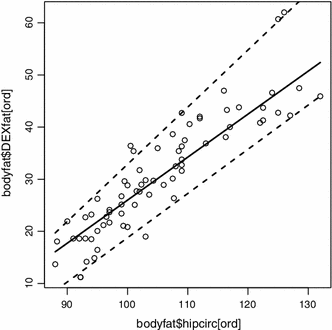Fig. 12

## Rights and permissions

Reprints and Permissions

## About this article

### Cite this article

Hofner, B., Mayr, A., Robinzonov, N. et al. Model-based boosting in R: a hands-on tutorial using the R package mboost . Comput Stat 29, 3–35 (2014). https://doi.org/10.1007/s00180-012-0382-5

Download citation

• Received:

• Accepted:

• Published:

• Issue Date:

### Keywords

• Boosting
• Component-wise functional gradient descent
• Generalized additive models
• Tutorial